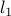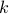# Recovery of Sparse Probability Measures via Convex Programming

• Authors: Mert Pilanci, Laurent El Ghaoui, and Venkat Chandrasekaran

• Status: In Proc. Advances in Neural Information Processing Systems (NIPS), December 2012.

• Abstract: We consider the problem of cardinality penalized optimization of a convex function over the probability simplex with additional convex constraints. The classicalregularizer fails to promote sparsity on the probability simplex sincenorm on the probability simplex is trivially constant. We propose a direct relaxation of the minimum cardinality problem and show that it can be efficiently solved using convex programming. As a first application we consider recovering a sparse probability measure given moment constraints, in which our formulation becomes linear programming, hence can be solved very efficiently. A sufficient condition for exact recovery of the minimum cardinality solution is derived for arbitrary affine constraints. We then develop a penalized version for the noisy setting which can be solved using second order cone programs. The proposed method outperforms known rescaling heuristics based on l1 norm. As a second application we consider convex clustering using a sparse Gaussian mixture and compare our results with the well known soft-means algorithm.

• Bibtex reference:

```@conference{PEC:12,
Author = {Mert Pilanci and Laurent {El Ghaoui} and Venkat Chandrasekaran},
Title = {Recovery of Sparse Probability Measures via Convex Programming},
Booktitle= {Proc. Advances in Neural Information Processing Systems ({NIPS})},
Year = {2012},
Month = dec
}
```